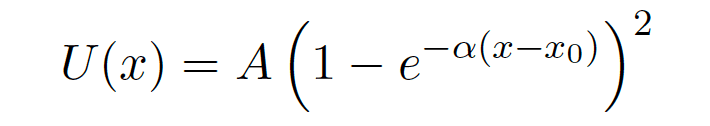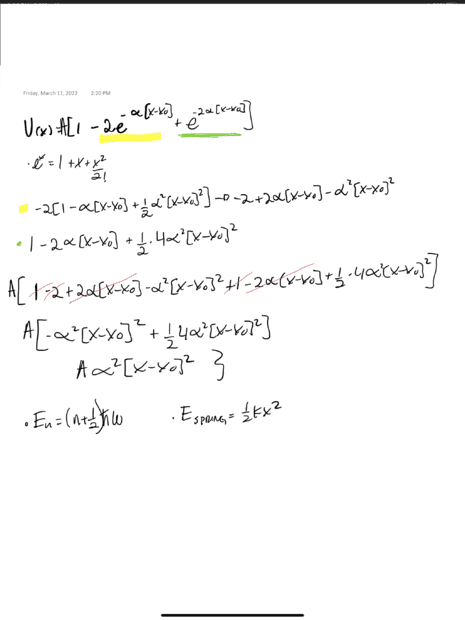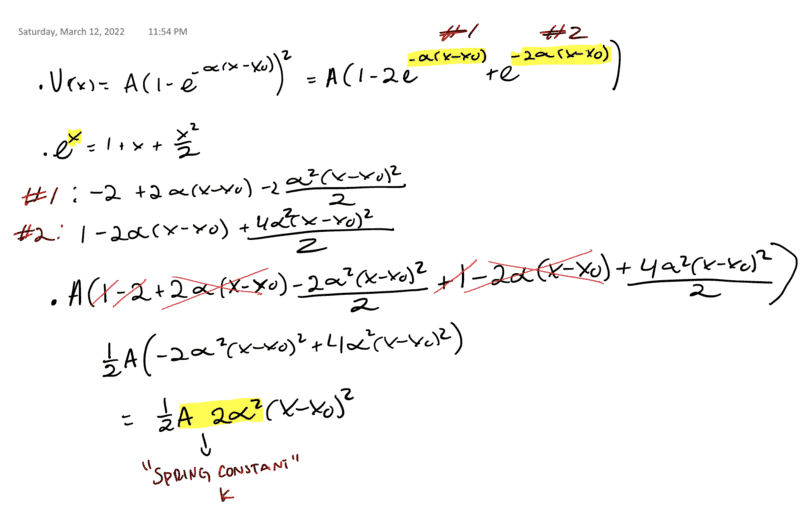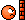# Finding the quantum number using Morse Potential

guyvsdcsniper
Homework Statement:
Using your result from the previous part, and what you know about harmonic oscillators, find an expression for the allowed energies as a function of the quantum number n. Not that since we have two atoms, the mass that shows up in your calculations should be the reduced mass μ=1/(1/m1+1/m2)
Relevant Equations:
U=1/2kx^2
Morse Potential
Taylor Series
E=(n+1/2)hbarw
This problem had me take the taylor series of the Morse Potential,until I got the first non zero term.

My result was U(x)=Aα2(x-x0)2.

I know to find the quantum number I can use En=(n+1/2)ℏω and I know I can relate that to the potential energy of a harmonic oscillator, 1/2kx2. So if this taylor series is similar to the PE of a H.O. I can just substitute that.

The problem is my taylor series is off by a factor of 1/2, so it doesn't resemble the PE of a Harmonic Oscillator.

Can anyone help me see where I am going wrong.

EDIT:

I rechecked my work and I think I was incorrect. Forgot the the 1/2! for my first taylor expansion. My apologies for anyone who viewed this already. I have edited my post.Last edited:

Homework Helper
2022 Award
Homework Statement:: Using your result from the previous part, and what you know about harmonic oscillators, find an expression for the allowed energies as a function of the quantum number n. Not that since we have two atoms, the mass that shows up in your calculations should be the reduced mass μ=1/(1/m1+1/m2)
Relevant Equations:: U=1/2kx^2
Morse Potential
Taylor Series
E=(n+1/2)hbarw

I know to find the quantum number I can use En=(n+1/2)ℏω and I know I can relate that to the potential energy of a harmonic oscillator, 1/2kx2. So if this taylor series is similar to the PE of a H.O. I can just substitute that.

The problem is my taylor series is off by a factor of 1/2, so it doesn't resemble the PE of a Harmonic Oscillator.
I don't understand the 1/2 discussion...you just redefine the constant. But there is a much bigger problem.
Your use of the correspondence to the Quantum oscillator is a good idea done badly. The value of the classical potential energy is essentially not related to the energy of the quantum eigenstrates. What does remain the same is the identification of ##\omega## from the respective equations. So what is the value of ##\omega##?

guyvsdcsniper
I don't understand the 1/2 discussion...you just redefine the constant. But there is a much bigger problem.
Your use of the correspondence to the Quantum oscillator is a good idea done badly. The value of the classical potential energy is essentially not related to the energy of the quantum eigenstrates. What does remain the same is the identification of ##\omega## from the respective equations. So what is the value of ##\omega##?
ω=##\sqrt{k/m}##.

BUt my Quantum Oscillator doesn't have ω in it, so how can I relate that to En?

I guess I had this approach because I did a problem very similar in class this week, where we were given m,k,and x for a spring and we were asked if we should worry about the quantum effects. So we set spring potential equal to the En=(n+1/2)ℏω

Homework Helper
2022 Award
BUt my Quantum Oscillator doesn't have ω in it, so how can I relate that to En?
You need to to identify the "spring constant" k for the Morse potential near its bottom. This has nothing to do with QM or ħ.
Once you have that you then know ##\omega=\sqrt {\frac k m}##

guyvsdcsniper
You need to to identify the "spring constant" k for the Morse potential near its bottom. This has nothing to do with QM or ħ.
Once you have that you then know ##\omega=\sqrt {\frac k m}##
I THINK I get what you are trying to say, so here is my attempt at identifying k.

Does this seem correct?Homework Helper
2022 Award
Looks good (I didn't check the Algebra). Remember that it is the "reduced mass" that appears in both the quantum and classical problem when written in the center of mass frame so I should have written ##~~\omega=\sqrt {\frac k {\mu}}##.

•guyvsdcsniper
guyvsdcsniper
Looks good (I didn't check the Algebra). Remember that it is the "reduced mass" that appears in both the quantum and classical problem when written in the center of mass frame so I should have written ##~~\omega=\sqrt {\frac k {\mu}}##.
So I ended up getting En=(n+1/2)ℏ##\sqrt{Aα^2/μ}##

Does that seem correct?

Homework Helper
2022 Award
I think that is what the problem is looking for. This is a little bit tricky depending upon how you define the zero of energy for the system. Also the Morse potential can be solved...but that is not introductory physics on any planet. You should (always) check that your result has the units of energy (I think it does)

•jim mcnamara
guyvsdcsniper
I think that is what the problem is looking for. This is a little bit tricky depending upon how you define the zero of energy for the system. Also the Morse potential can be solved...but that is not introductory physics on any planet. You should (always) check that your result has the units of energy (I think it does)
I think that does make sense as well. But then I run into a problem for the next question which states

"Calculate the wavelength of a photon emitted from diatomic hydrogen as it transitions from the third excited
state to the ground state. For this molecule, A = 4.787 eV and = 5:396 10^-11 m.""

Well if En= (n+##\frac{1}{2}##)ℏω, and from the third state to ground state (0), I should get En=ℏω

And I know E = ##\frac{hc}{λ}## I can solve for lambda and get λ=##\frac{hc}{ℏω}## .

I think that makes sense, but I don't have a value for the masses when I express omega in terms of k and μ. Mass should play a factor right? My teacher relies on us approximating values at times, so I might need to do that, but from your perspective, does this seem like the right approach?

Homework Helper
2022 Award
Diatomic hydrogen !
mH=1amu
And how do you get 3-0=1? (n=3 and n=0)

guyvsdcsniper
Diatomic hydrogen !
mH=1amu
And how do you get 3-0=1? (n=3 and n=0)
Crap I totally looked that over in the question. Sorry. Your right, It is diatomic hydrogen.

Well just looking at the (n+##\frac{1}{2}##) part of the En= (n+##\frac{1}{2}##)ℏω,

if n = 3 then I get ##\frac{3}{2}## and at ground state, n=0, so i get -##\frac{1}{2}## which adding together gets me 1.

guyvsdcsniper
Crap I totally looked that over in the question. Sorry. Your right, It is diatomic hydrogen.

Well just looking at the (n+##\frac{1}{2}##) part of the En= (n+##\frac{1}{2}##)ℏω,

if n = 3 then I get ##\frac{3}{2}## and at ground state, n=0, so i get -##\frac{1}{2}## which adding together gets me 1.
EDIT: at n=0 i get 1/2, but the difference between states gives me 1.

Homework Helper
2022 Award
1)Attach brain.
2)plug n=3 into equationguyvsdcsniper
1)Attach brain.
2)plug n=3 into equation. Let's act like I never wrote my last post.

•hutchphd
•